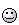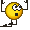# 2 Capacitors and energy density

• Shreya
In summary, the conversation discusses three arguments for determining which of two parallel plate capacitors, one with double the plate area of the other but the same width and equal charge, has more energy. Argument 1 states that the capacitor with more volume must have more energy, according to the first equation. Argument 2 points out that, according to the second equation, the capacitor with higher capacitance must have lesser energy. Argument 3 argues that the capacitor with same width and electric field must have more energy, according to the third equation. After considering an extreme case and using the formula for capacitance, it is concluded that the capacitor with smaller plate area has more energy due to the inverse relationship between electric field and plate area in the first equation

#### Shreya

Homework Statement
Lets consider 2 parallel plate capacitors ##C_1## and ##C_2##.
##C_1## has plate area twice of the ##C_2##, but same width. If both stores equal charge ##Q##. Which one has more energy?
Relevant Equations
$$U = (Volume) \frac 1 2 \epsilon E^2$$
$$U= \frac {Q^2}{2C}$$
$$U= \frac 1 2 CV^2$$
I developed three arguments to answer this question. Argument no 2 seems to be wrong, but I cant figure out why. I know one/more of my arguments are flawed. Please be kind to help me figure this out.

Argument 1) Since they have same charge on them, the ##E## between them must be same. The one with more volume ##C_1## must have more energy, according to the first equation.

Argument 2) According to equation 1, ##C_1## must have lesser Energy that ##C_2##, due to higher capacitance. (Refer second equation)

Argument 3) Due to same width and same ##E##. they must have same ##V## between them. According to equation 3, ##C_1## must have more Energy

Shreya said:
Homework Statement:: Lets consider 2 parallel plate capacitors ##C_1## and ##C_2##.
##C_1## has plate area twice of the ##C_2##, but same width. If both stores equal charge ##Q##. Which one has more energy?
Relevant Equations:: $$U = (Volume) \frac 1 2 \epsilon E^2$$
$$U= \frac {Q^2}{2C}$$
$$U= \frac 1 2 CV^2$$

I developed three arguments to answer this question. Argument no 2 seems to be wrong, but I cant figure out why. I know one/more of my arguments are flawed. Please be kind to help me figure this out.

Argument 1) Since they have same charge on them, the ##E## between them must be same. The one with more volume ##C_1## must have more energy, according to the first equation.

Argument 2) According to equation 1, ##C_1## must have lesser Energy that ##C_2##, due to higher capacitance. (Refer second equation)

Argument 3) Due to same width and same ##E##. they must have same ##V## between them. According to equation 3, ##C_1## must have more Energy
By 'width' I assume you mean plate-separation (##d##).

To get an intuition of what’s going on (and why two of the arguments are wrong) you could try considering a more extreme case. Let '##A##' be plate area. Suppose ##A_1 = 1000,000A_2##. Can you see what will happen to the magnitude of the electric fields?

Alternatively (or even better, additionally) do yo know the formula for ##C## in terms of ##A, d## and any constant(s)? If so, can you use this formula to help?

•Shreya
1) Considering the extreme case, I can see that ##E## of ##C_1## must be small, since the same charge will be spread over a larger area ##E = \frac \sigma \epsilon##. And since ##E## is squared (unlike Volume(##=Ad##) in Equation 1, low ##E## would mean lower ##U##.

$$C= \epsilon \frac {A}{d}$$
2) I know this formula for Capacitance of a Parallel plate capacitor. So greater area would mean greater ##C## and therefore lower ##U##.

3)Lower ##E## would mean lower potential drop for the same plate seperation. And since ##V## is squared, its effect overrides that of increase in C, implying lower Energy.

@Steve4Physics, I have modified my arguments using your suggestion. Could you please verify them.

Shreya said:
@Steve4Physics, I have modified my arguments using your suggestion. Could you please verify them.
Yes. All the arguments are now correct (and give the same conclusion!).

You might consider slightly more detail. For example for 1) you could note:
a) volume ##\propto A##;
b) ##E \propto \frac 1A##;
c) so ##U \propto A(\frac 1{A^2}) \propto \frac 1A##.

Doing the maths along with the qualitative analyse is very useful. For example, you may be able to understand (qualitatively) that some quantity Y decreases when quantity X increases, but without the proper analysis you won’t know the precise relationship, e.g. is ##Y \propto \frac 1X## or is ##Y \propto \frac 1{X^2}## or something else?.

To derive the equation for ##U## in terms of ##\epsilon , A, d## and ##Q## is straightforward. The dependence of ##U## on ##A## (for fixed ##Q## and ##d##) will then be very clear.

By the way, another intuition into the problem is to note that to put a charge onto a small area requires more work than putting the same onto a larger area – because of the additional work needed to overcome the mutual repulsion between the charge-elements.

•Shreya
Steve4Physics said:
You might consider slightly more detail. For example for 1) you could note:
a) volume ∝A;
b) E∝1A;
c) so U∝A(1A2)∝1A.

Doing the maths along with the qualitative analyse is very useful. For example, you may be able to understand (qualitatively) that some quantity Y decreases when quantity X increases, but without the proper analysis you won’t know the precise relationship, e.g. is Y∝1X or is Y∝1X2 or something else?.
That makes sense. Doing such an analysis for 2 & 3 also yields the above result!I will make sure that I do such analysis from now.
Steve4Physics said:
To derive the equation for U in terms of ϵ,A,d and Q is straightforward. The dependence of U on A (for fixed Q and d) will then be very clear.
That would be: $$U = \frac {Q^2d}{2 \epsilon A}, which confirms what we discussed above. Steve4Physics said: By the way, another intuition into the problem is to note that to put a charge onto a small area requires more work than putting the same onto a larger area – because of the additional work needed to overcome the mutual repulsion between the charge-elements. Yes! Didnt realise that until now! Thank you so much @Steve4Physics for helping me through this problem. I appreciate that you made me think and arrive at the solution, instead of solving it for me. Thank you!•Steve4Physics In comparison problems of this kind it is useful to write equations for the quantities that you are comparing in terms of the quantities that are given. Let me explain. You want to compare stored energies ##U##. Using ##Q=CV##, you can write the stored energy in three different ways each of which has only two of the variables, ##Q##, ##C## and ##V##:$$\begin{align} & U=\frac{1}{2}CV^2 \\ & U=\frac{Q^2}{2C} \\ & U =\frac{1}{2}QV.\end{align}$$Which one to use? Look at what is given, ##C_2 = 2C_1 \implies## if ##C_1=C## then ##C_2=2C.## ##Q_1=Q_2=Q.## It should be obvious that you should use equation (2) that has capacitance and charge on the right-hand side. Then you get,$$\begin{align} & U_1=\frac{Q_1^2}{2C_1}=\frac{Q^2}{2C} \nonumber \\ & U_2=\frac{Q_2^2}{2C_2}=\frac{Q^2}{2\times 2C}= \frac{Q^2}{4C}=\frac{1}{2}U_1\nonumber \end{align}Now you can clearly see which of the stored energies is greater. As long as one capacitor has twice the capacitance of the other, this result is true and independent of how the capacitors are shaped or what the electric fields look like.

••Steve4Physics and Shreya
Thanks a lot @kuruman ! I think this insight can be extended to other formulae as well, which is awesome.

•kuruman